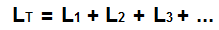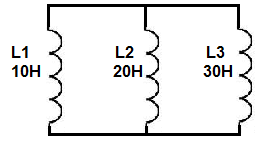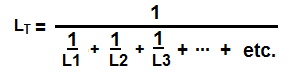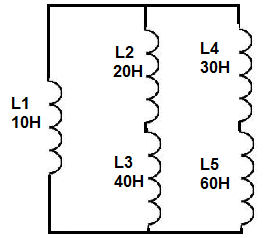﻿ Inductors in Series and in Parallel ﻿# Inductors in Series and in ParallelWe will go over the mathematical formulas for calculating series and parallel inductance so that we can compute the total inductance values of actual circuits.

### Inductors in Series

Inductors in series are inductors that are placed back-to-back.

Below is a circuit where 3 inductors are placed in series.You can see the inducotrs are in series because they are back-to-back against each other. The best way to think of a series circuit is that if current flows through the circuit, the current can only take one path. You can see in the above circuit that if current flowed through it, it could only take one path.

### Formula for Adding Inductors in Series

The formula to calculate the total series inductance of a circuit is:So to calculate the total inductance of the circuit above, the total inductance, LT would be:So using the above formula, the total inductance is 60H.

Note- When inductors are in series, as the formula shows, they simply add together. Thus, the total inductance of a series circuit will always be greater than any of the individual inductor values.

### Inductors in Parallel

Inductors in parallel are inductors that are connected side-by-side in different branches of a circuit.

Below is a circuit where 3 inductors are in parallel:You can see that the inductors are in parallel because they are all on their own separate branches in the circuit. The best way to think about parallel circuits is by thinking of the path that current can take. When current is travelling through a parallel circuit, the current can take various paths through the circuits, such as to go through any of the branches containing the inductors. In series, this is not the case. Current can only take one path.

### Formula for Adding Inductors in Parallel

The formula to calculate the total parallel inductance is:So to calculate the total inductance of the circuit above, the total inductance, LT would be:So using the above formula, the total inductance is 5.45Ω.

Note- When inductors are in parallel, the total inductance value is always less than the smallest inductor of the circuit. In other words, when inductors are in parallel, the total inductance shrinks. It's always less than any of the values of the inductors.

### Inductor Circuit in Series and In Parallel

We'll now do an inductor circuit in which inductors are both in series and in parallel in the same circuit.

Below is a circuit which has inductors in both series and parallel:So how do we add them to find the total inductance value?

First, we can start by finding the resistance of the resistors in series. In the first branch, containing the 20H and 40H inductors, the series resistance is 60H. And in the second branch, containing the 30H and 60H inductors, the series inductance is 90H. Now in total, the circuit has 3 inductances in parallel, 10H, 60H, and 90h. Now, we plug these 3 values into the parallel inductance formula and we get a total inductance of 7.83H.

If you want to test the above series and parallel connections out practically, get 1mH inductor or whatever inductors you have, but let them be of the same value. In this example, I'll stick with 2 1mH inductors. Take the inductors and place them in series. Now take a multimeter and place the multimeter in the inductance setting (if available) and place the probes over the 2 inductors You should read just about 2mH, which is double the value of both inductors. This proves that inductors add when connected in series. Now place the inductors in parallel. Take the multimeter probes and place one end on one side of a inductor (either one) and place the other probe on the other side of that inductor. You should now read about 0.5mH, or half the value, because inductance decreases in parallel. This is a practical, real-life test you can do to show how inductors add.

If you have a circuit where you would like to calculate the series or parallel inductance, check out our online Parallel and Series Inductor Calculator. This allows you to calculate the total inductance value of circuits.

Related Resources